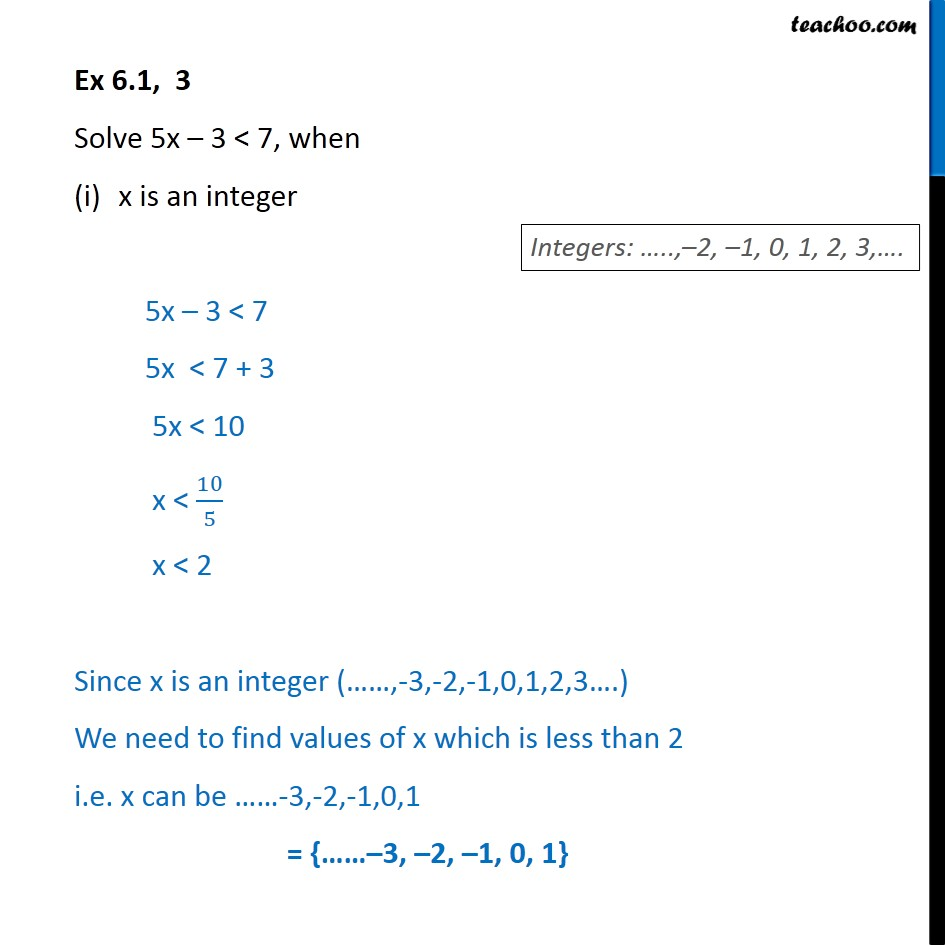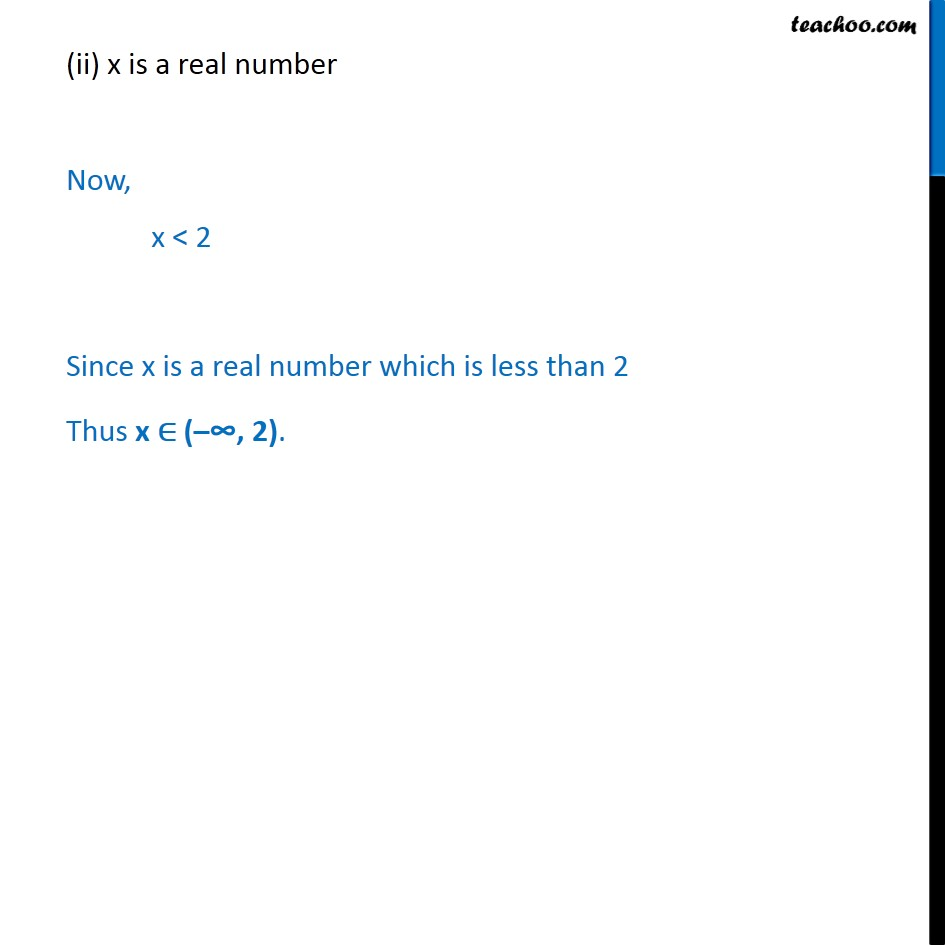1. Chapter 6 Class 11 Linear Inequalities (Term 2)
2. Serial order wise
3. Ex 6.1

Transcript

Ex 6.1, 3 Solve 5x 3 < 7, when x is an integer 5x 3 < 7 5x < 7 + 3 5x < 10 x < 10 5 x < 2 Since x is an integer ( ,-3,-2,-1,0,1,2,3 .) We need to find values of x which is less than 2 i.e. x can be -3,-2,-1,0,1 = { 3, 2, 1, 0, 1} (ii) x is a real number Now, x < 2 Since x is a real number which is less than 2 Thus x ( 2).